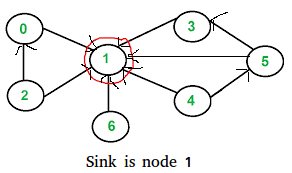GFG App
Open AppBrowser
Continue

# GATE | Gate IT 2005 | Question 88

Q84 Part_A
A sink in a directed graph is a vertex i such that there is an edge from every vertex j ≠ i to i and there is no edge from i to any other vertex. A directed graph G with n vertices is represented by its adjacency matrix A, where A[i] [j] = 1 if there is an edge directed from vertex i to j and 0 otherwise. The following algorithm determines whether there is a sink in the graph G.
```i = 0
do {
j = i + 1;
while ((j < n) && E1)
j++;
if (j < n) E2;
} while (j < n);

flag = 1;
for (j = 0; j < n; j++)
if ((j! = i) && E3)
flag = 0;

if (flag)
printf("Sink exists");
else
printf ("Sink does not exist");
```

Choose the correct expressions for E1 and E2

(A) E1 : A[i][j] and E2 : i = j;
(B) E1 : !A[i][j] and E2 : i = j + 1;
(C) E1: !A[i][j] and E2 : i = j;
(D) E1 : A[i][j] and E2 : i = j + 1;

Explanation: For vertex i to be a sink, there should be no edge from i to any other vertex.According the input given in question,

```A[i][j] = 1 means there is an edge from vertex i to j.
A[i][j] = 0 means there is no edge from i to j
```

For a node to i to be sink,

```A[i][j] should be 0 for all j
A[j][i] should be 1 for all j.```

The above pseudo code checks every vertex i for sink, starting from i = 0. It basically checks every vertex j after i for being a sink. The trick in pseudo code is, it doesn’t check for j smaller than i. The i picked by this loop may not be sink. It basically makes sure that we don’t ignore a potential sink. The check whether i is actually a sink or not is done later after do while loop.

Vertex i is a potential sink while A[i][j] is zero
Thus, E1 : !A[i][j]

If the above condition is false, then i is not a sink. All j < i can also not be a sink because there is no edge from i to j.
Now, the next potential sink can be j.
So, E2 : i = j

Thus, option (C) is correct.

My Personal Notes arrow_drop_up